An electric kettle takes 4 A current at 220 V. How much time will it take to boil 1 kg of water from temperature ${20}^{°}$C? The temperature of boiling water is ${100}^{°}$C

(a) 6.3 min

(b) 8.4 min

(c) 12.6 min

(d) 4.2 min

High Yielding Test Series + Question Bank - NEET 2020

Difficulty Level:

A cell can be balanced against 110cm and 100 cm of potentiometer wire, respectively with and without being short-circuited through a resistance of . Its internal resistance is

(a) 1.0 $\Omega$

(b) 0.5 $\Omega$

(c) 2.0 $\Omega$

(d) zero

Concept Questions :-

Grouping of cells
High Yielding Test Series + Question Bank - NEET 2020

Difficulty Level:

In the circuit shown, the current through the 4 $\Omega$resistor is 1 A when the points P and M are connected to a DC voltage source. The potential difference  between the points M and N is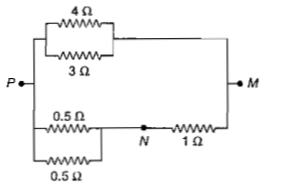1. 1.5 V

2. 1.0 V

3. 0.5 V

4. 3.2 V

Concept Questions :-

Combination of resistors
High Yielding Test Series + Question Bank - NEET 2020

Difficulty Level:

A wire of a certain material is streched slowly by ten per cent. Its new resistance and specific resistance become respectively

(a) 1.2 times, 1.1 times

(b) 1.21 times,same

(c) both remain the same

(d) 1.1 times, 1.1 times

High Yielding Test Series + Question Bank - NEET 2020

Difficulty Level:

A current of 3A flows through the 2$\Omega$ resistor shown in the circuit. The power dissipated in the $5\Omega$ resistor is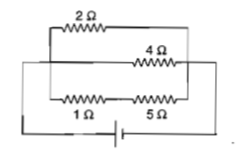(a) 4 W                                              (b) 2 W

(c) 1 W                                              (d) 5 W

High Yielding Test Series + Question Bank - NEET 2020

Difficulty Level:

See the electrical circuit shown in this figure. Which of the following equation is a correct equation for it?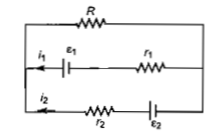1. ${\epsilon }_{1}-\left({i}_{1}+{i}_{2}\right)R-{i}_{1}{r}_{1}=0$

2. ${\epsilon }_{2}-{i}_{2}{r}_{2}-{\epsilon }_{1}-{i}_{1}{r}_{1}=0$

3. $-{\epsilon }_{2}-\left({i}_{1}+{i}_{2}\right)R+{i}_{2}{r}_{2}=0$

4. ${\epsilon }_{1}-\left({i}_{1}+{i}_{2}\right)R+{i}_{1}{r}_{1}=0$

Concept Questions :-

Kirchoff's voltage law
High Yielding Test Series + Question Bank - NEET 2020

Difficulty Level:

A student measures the terminal potential difference (V) of a cell (of emf $\epsilon$ and internal resistance r) as a function of the current (I) flowing through it. The slope and intercept of the graph between V and I, then respectively, equal :

(a)                                (b)

(c)                                (d)

Concept Questions :-

Emf and terminal voltage
High Yielding Test Series + Question Bank - NEET 2020

Difficulty Level:

A wire of resistance  is bent to form a complete circle of radius 10 cm. The resistance between its two diametrically opposite points, A and B as shown in the figure, is :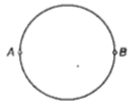(a)                                                (b)

(c)                                                   (d)

Concept Questions :-

Combination of resistors
High Yielding Test Series + Question Bank - NEET 2020

Difficulty Level:

Consider the following two statements :

(A) Kirchhoff's junction law follows from the conservation of charge.

(B) Kirchhoff's loop law follows from the conservation of energy.

Which of the following is correct?

(a) Both (A) and (B) are wrong

(b) (A) is correct and (B) is wrong

(c) (A)  is wrong and (B) is correct

(d) Both (A) and (B) are correct

Concept Questions :-

Kirchoff's voltage law
High Yielding Test Series + Question Bank - NEET 2020

Difficulty Level:

A potentiometer circuit is set up as shown.The potential gradient across the potentiometer wire, is k volt/cm and the ammeter, present in the circuit, reads 1.0A when two way key is switched off. The balance points, when the key between the terminals (i) 1 and 2 (ii) 1and 3, is plugged in, are found to be at lengths  respectively.The magnitudes, of the resistors R and X, in ohm, are then, equal, respectively, to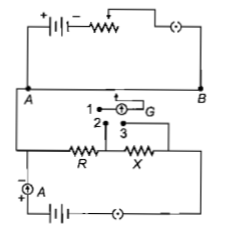(a)

(b)

(c)

(d)

Concept Questions :-

Meter bridge and potentiometer